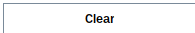Superellipsoid - Maple Help

Superellipsoid

Main Concept

A superellipsoid is a 3-dimensional solid whose horizontal cross-sections are superellipses with the same exponent r, and whose vertical cross-sections through the centre are superellipses with the same exponent t. The general implicit equation for a superellipsoid is

${\left({\left|\frac{x}{A}\right|}^{r}+{\left|\frac{y}{B}\right|}^{r}\right)}^{\frac{t}{r}}+{\left|\frac{z}{C}\right|}^{t}\le 1$.

The parameters t and r are positive real numbers which control the amount of flattening at the tips and equator of the solid. Factors A, B, and C scale the basic shape along each axis and are called the semi-diameters of the solid.

If , the equation for a superellipsoid becomes a special case of the superquadric equation.Interesting Solids When , the horizontal cross sections of the solid are circles, so the superellipsoid is a solid of revolution: it can be obtained by rotating a superellipse of exponent t around the vertical axis. When , the solid is an ordinary ellipsoid. In particular, if , the solid is a sphere of radius A. When  and , the superellipsoid is a special solid known as Piet Hein's "superegg".

The following graph shows a superellipsoid. Use the sliders to adjust the semi-diameters and parameters to see what solids you can make.

 Parameter, tParameter, rSemi-Diameter, ASemi-Diameter, BSemi-Diameter, CMore MathApps